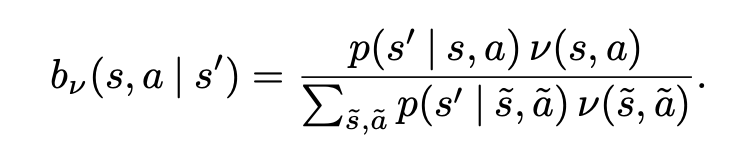## The paper: Provably efficient RL with Rich Observations via Latent State Decoding

Key Idea: They create a mapping from observations to latent states, and use that mapping for exploration policies.

They mention these works which have good exploration strategies and work in tabular settings:

• Near-optimal reinforcement learning in polynomial time
• R-max-a general polynomial time algorithm for near-optimal reinforcement learning

The challenges is to have these methods “adapt to rich observation spaces”

The number of interactions required by these methods are polynomial in # of observed states.

“In order to avoid the dependency on the observation space, one must exploit some inherent structure in the problem.”

Describe works where they assume that observations are generated from a small number of latent states and assume that this smaller number (which would reduce the hardness of the problem) could be implicitly learned.

This work strives to learn that latent state explicitly with the use of a decoding function. Which would reduce the problem to a similar one as the tabular setting.

## They present two key ideas:

• block Markov Decision Process
• ε-policy cover

“The main challenge in learning the decoding function is that the hidden states are never directly observed. Our key novelty is the use of a backward conditional probability vector (Equation 1) as a representation for latent state, and learning the decoding function via conditional probability estimation, which can be solved using least squares regression.”

Backward conditional probability vector (see first image at bottom of post)

• A backward probability is the probability of a specific previous state and previous action given the current state. (Opposite of transitions, where we predict the next state given current state and action.)

## Block Markov Decision Process

The environment has three main parts

## 03. An observable context space X

It can then be described in terms of the state-transition function and context-emission function:

## p(s’|s,a) & q(x|s)

The first, p, predicts the next latent state given the current state and action, and the second, q, gives the context space given the current latent state.

The block structure is then defined as such:

“Each context x uniquely determines its generating state s. That is, the context space X can be partitioned into disjoint blocks Xs, each containing the support of the conditional distribution q(· | s).”